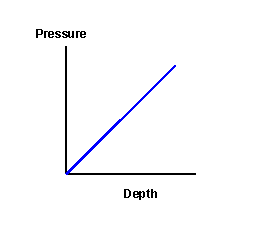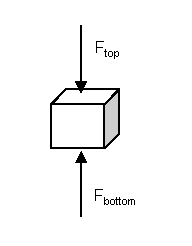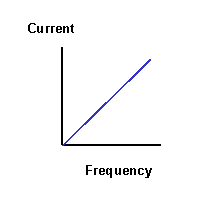# Solutions to SQA examination

### Paper II Solutions

```
1.a.i.	u = 0m/s			s = ut + 1/2at2
a = 4m/s2			s = 0x7 + 1/2x4x72
t = 7s
s = ?				s = 98m

a.ii. First Test
To calculate the final speed in the first test(v1)use:v1 = u1 + at.

=>v1 = 0 + 4x7
=>v1 = 28m/s

Second Test
u2 = 0m/s		v22 = u22 + 2as
a = 4m/s2		v22 = 02 + 2x4x196
s = 196m		v22 = 1568m
v2 = ?			v2 = 39.6m/s

Increase in speed = v2 - v1
Increase in speed = 39.6m/s - 28m/s
Increase in speed = 11.6m/s

a.iii.	Third Test
u = 40m/s			v2 = u2 + 2as
a = -2.5m/s2			=>s = (v2 - u2)/2a
v = 0m/s			=>s = (02-402)/2x-2.5
s = ?				=>s = -1600/-5
=>s =  320m

b.i.To calculate the acceleration of the trolley the student needs to measure the:
length of the card(d);time it takes the trolley to travel from the first light gate to the second(t1).

The computer must measure the time the card cuts the light beam produced by the
light gate at:
the top of the slope(t2);the bottom of the slope(t3).

b.ii.	The speed at the top of the slope(u) is calculated using:
u=d/t2

The speed at the bottom of the slope(v) is calculated using:
v=d/t3

The acceleration is calculated using:

a=(v-u)/(t1)

2.a.	The bungee rope is at its maximum length at 3.6s. At this time the sign of
the velocity of the bungee jumper changes, indicating a change in their direction
from down to up.

b.	To calculate the unbalanced force acting on the jumper, the jumper's acceleration
must be calculated and then Newtons second law of motion applied.

u = -18m/s		a = (v-u)/t
v =  16m/s		a = (16--18)/3
t =  3s			a = 34/3
a = ?			a = 11.33m/s2

[N2] F = ma
F = 55x11.33
F = 623.3N

c.	An elastic rope must be used to ensure the change in velocity does not take place
over a very short time interval. If the time interval was short the acceleration of
the jumper and hence the unbalanced force acting on the jumper would be much greater.
This force could be large enough to injure the jumper.

3.a.	Total momentum before Pbefore = Total momentum after Pafter

b.i.	Change in momentum of vehicle A(DP(A)) = P(A)after-P(A)before
Change in momentum of vehicle B(DP(B)) = P(B)after-P(B)before

DP(A) = MA(VA-UA)
DP(A) = 0.75(0.82-0.4)
DP(A) = 0.315kgm/s

DP(B) = MB(VB-UB)
DP(B) = 0.5(0-0.63)
DP(B) = -0.315kgm/s

Notes: 	i. Vector quantities to the right have been assigned positive values.
ii.Vector quantities to the left must be assigned negative values.

The above calculations therefore show that the change in momentum of vehicle A
is equal and opposite to that of vehicle B.

b.ii.	If kinetic energy is conserved the collision is elastic, and if it is not
the collision is inelastic.

Ekbefore = 1/2MAUA2 + 1/2MBUB2
Ekbefore = 0.5X0.75X0.822 + 0.5X0.5X02

4.a.b.i.	The lower surface area of the submarine will experience a greater pressure
than the top surface because it is a greater depth below the surface.
This pressure difference means that there will also be a greater force
exerted on the lower surface than the top surface as the force(F) on each
surface is equal to the product of the pressure(p) and area(A).

Fupthrust = Fbottom - Ftopii.	mass = density x volume
mass = 1.02x103 x 14.5
mass = 14790Kg

iii.	The effective mass of the submarine is decreased when water in the tanks is
replaced with air, however, the upthrust force does not change. Therefore, as the
acceleration is calculated using newtons second law, a = Fupthrust/m, the acceleration
increases as mass decreases.

5.a.	When the bridge is balanced:
Rvariable/Rthermistor = R1/R2

Where 	R1 = 500W
R2 = 900W
Rvariable = 450W

Rthermistor = (RvariablexR2)/R1
Rthermistor = (450x900)/500)
Rthermistor = 810W

b.i.	Mean = Total/Number of readings
Mean = (852+854+848+851+853)/5
Mean = 4258/5
Mean = 851.6W

b.ii.	Random Error = (max-min)/Number of readings
Random Error = (854-848)/5
Random Error = 6/5
Random Error = 1.2W

c.	A systematic error is one that will make all measurements either too high or too
low.

6.a.	Vlost = emf - (V1+V2)
Vlost = 1.60 - (1.20 + 0.30)
Vlost = 0.10V

b.	Vlost = Ir
r = Vlost/I
r = 0.1/0.04
r = 2.5W

c.i.	I = emf/(R1+R2+r)

Where 	R1 = 30
R2 = Variable resistor
r = 2.5W

=>IR1 + IR2 + Ir = emf
=>IR2 = emf - IR1 - Ir
=>R2  = (emf - IR1 - Ir)/I
=>R2  = (1.60 - 0.02x30 - 0.02x2.5)/0.02
=>R2  = 0.95/0.02
=>R2  = 47.5W

c.ii.	Vtpd = emf - Vlost
Vlost = Ir

By decreasing the value of the variable resistor the current in the circuit increases.
Consequently, the "lost volts"  increase, leading to a drop in the Vtpd.

7.a.i.	Ipeak = 1.414Irms
Ipeak = 1.414x200
Ipeak = 282.8mA

a.ii.b.i	To calculate the charge stored on the capacitor, when the current is 2mA, the
voltage across the capacitor (Vc) must be calculated and then the equation
Q = CVc used.

Vsupply = Vc + Vresistor
Vc = Vsupply - Vresistor

Vresistor = IR
Vresistor = 0.002x4000
Vresistor = 8V

Vc = 12 - 8
Vc = 4V

Q = CVc
Q = 5000x10-6x4
Q = 0.02C

b.ii.	When the capacitor is fully charged the potential difference across it is 12V.

E = CV2/2
E = 5000x10-6x122/2
E = 0.360J

8.a.i.	The amplifier is acting in inverting mode. The gain is therefore given by the equation:

Voutput/Vinput = -Rfeedback/Rinput
Voutput = -VinputxRfeedback/Rinput
Voutput = -0.20x400/22

Voutput = 3.64V

a.ii.	The theoretical output voltage with a 10MW feedback resistor is calculated as above.

Voutput = -VinputxRfeedback/Rinput
Voutput = -2.0x10000/22
Voutput = -909.1V

However, the maximum output voltage from the amplifier is limited by the voltage
supplied to the amplifier. In this case the the output voltage is limited to a range
of -13V to +13V, therefore the output voltage would be -13V.

b.i.	The amplifier is operating in differential mode.
b.ii.	When there is no difference between the voltages input into the inverting input(V-)
and non inverting input(V+) the output voltage(Voutput) will be 0V.
At this voltage the technician knows the light sources are equally bright.

b.iii.V- = 1.65V
V+ = 1.85V

Vouput = -(Rfeedback/R1)x(V--V+)
Vouput = -(100/10)x(1.65-1.85)
Vouput = -10x-0.20
Vouput = -2.0V

b.iv.	The output voltage will:
become less negative as V- approaches 0.85V;be equal to 0V when V- is equal to 0.85V;become positive when V- is greater than 1.85V.
The above statements can be explained by reference to the equation:
Vouput = -(Rfeedback/R1)x(V--V+).

As V- increases the difference between V- and V+ changes.
The affect of this is as described above.

9.a.	qcritical = sin-1(1/nwater)
qcritical = sin-1(1/1.33)
qcritical = sin-10.752
qcritical = 48.75o

As the incident angle is greater than the critical angle the light is
totally internally reflected.

b.	The refractive index of the new material is equal to the gradient of the graph.

n = 0.4/0.28
n = 1.43	(taking the answer to two decimal places)

c.	lliquid = lair/n
lliquid = 670nm/1.47
lliquid = 455.8nm

10.a.i.	dsinq = nl

d = 5.0x10-6m
q = 14o
n = 2
l = ?

l = dsinq/n
l = 5.0x10-6sin14o/2
l = 6.04x10-7m

a.ii.	By decreasing the slit separation to 2.0x10-6m the distance between maxima,
as well as angle q, will increase. This will result in the measurement errors
producing smaller percentage errors, and therefore lead to a more accurate value of l.

b.i.	White light is made up of many different wavelengths of light. At the position of the
central maximum there is no path difference between any pair of waves of a particular
wavelength. These waves are in phase and interfere constructively to produce a bright
band of white light.

b.ii.	All other maxima are in the form of a continuous spectra because constructive
interference, of each wavelength produced by the source, takes place at a different
position on the screen. This leads to a bright band of each colour where that
wavelength is interfering constructively.

11.a.i.	The addition of group III or V atoms to pure semiconductor is called doping.

a.ii.Doped semiconductor has a lower resistance.

b.i.	The diode is operating in photoconductive mode.

b.ii.	Photons incident on the pn junction provide enough energy to excite electrons
into the conduction band and leave holes in the valence band. The intensity of light
determines the number of charge carriers produced. At low intensity fewer charge carriers
are produced resulting in a smaller current flowing in the circuit for a given applied
potential difference.

c.	E = hf	f = c/l

E = hc/l
l = hc/E
l = (6.63x10-34x3x108)/2.3x10-19
l = 864.8nm

12.a.	To conserve mass number and atomic number the emmitted radiation must be beta(b).
b = 0-1e

b.i.	D = E/m
E = Dm
E = 750x10-6x0.04
E = 3x10-5J or 30mJ

b.ii.H/t = QxD/t
12.5x10-6 = Qx750x10-6/120
Q = 12.5x10-6x120/750x10-6
Q = 2

c.	Number of half value thicknesses(N(t1/2)) = Total thickness/half value thicknesses(t1/2)
N(t1/2) = 24/8
N(t1/2) = 3

t1/2     t1/2    t1/2
1200 --> 600 --> 300 --> 150

The new count rate is 150cpm.